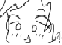Math Is Fun Forum

Discussion about math, puzzles, games and fun.   Useful symbols: ÷ × ½ √ ∞ ≠ ≤ ≥ ≈ ⇒ ± ∈ Δ θ ∴ ∑ ∫  π  -¹ ² ³ °

You are not logged in.

#1 2019-05-22 06:52:29

thefurlong
Member
Registered: 2019-05-22
Posts: 1

Binomial Coefficients in terms of Roots of Unity

I found an interesting identity relating Binomial Coefficients to Roots of Unity.  I wonder if anybody knows anything else about it.

Start with Lagrange Interpolation.  This is a way of constructing a polynomial from

points, and their values.  Suppose I have a function,
, which I know at unique points,
.

Then, Lagrange Interpolation gives us the interpolating polynomial:

In other words, you obtain it by adding a bunch of polynomials that are guaranteed to be 0 at every sample point, but the one selected point.  Each such polynomial term is then normalized to evaluate to the function at that selected point.  Each such polymomial term is known, unsurprisingly, as a basis term.

This polynomial is unique, in that there are no other polynomials of degree

, which also evaluates to the same value at those points.  That's because, as long as the sample points are unique, the corresponding Vandermonde matrix (https://en.wikipedia.org/wiki/Vandermonde_matrix) is nonsingular.

On the other hand, binomial expansion equates the two polynomials:

So, I could pick any n points I want, and if I evaluate

, where
is in [0,n], then the corresponding Lagrange interpolation, when fully expanded will have the same coefficients as that in the binomial expansion.

This suggests we can use this to find interesting identities of binomial coefficients.

My first attempt at this is to sample at the roots of unity for degree n, since roots of unity are really well behaved.  In fact, let

be the polynomial, such that
, where
is the kth root of unity.

Long Polynomial Division shows that this is equivalent to,

Then the corresponding basis polynomial will be,

Collecting coefficients, we get,

or

where

So, my question is: is anyone familiar with this, or if it is useful for anything?

I would also like to share that I am considering modifying this, so that I am working with

, where
approaches 0, and see what happens.

---

Update:

There is another way to see why this works out.

Recall that a sum of powers is given by

, if
is not 1.

In the case of roots of unity, if I take all roots of unity, and raise each one to a fixed power, say q, and sum them, I will get 0, because of the above identity, unless, of course, q is, itself 0.

In that case, I will simply get

(this is the principle behind the Discrete Fourier Transform, btw).

So, in that equation, what is happening is that the

exponent is "selecting" the correct binomial coefficient by evaluating to
everywhere but that coefficient, and to
, at the coefficient.  And, of course, the
in the denominator normalizes things, so you get the bare binomial coefficient.

Very interesting...

Last edited by thefurlong (2019-05-22 12:51:32)

Offline

#2 2019-05-22 13:31:57

Mathegocart
MemberRegistered: 2012-04-29
Posts: 2,199